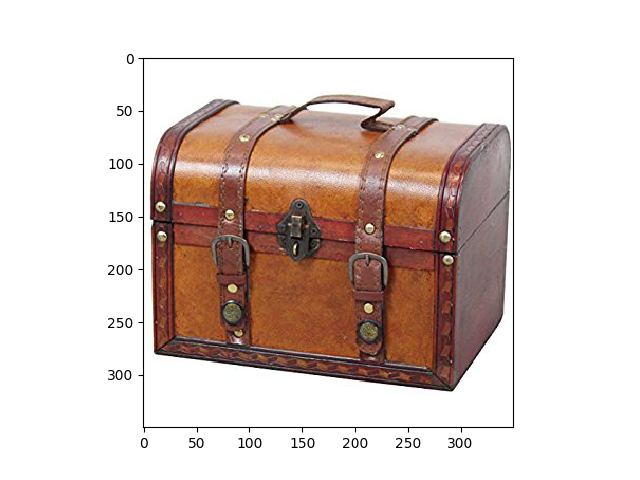# Pipeline using Time Series Padding and Truncation¶

This is a basic example using the pipeline to learn a feature representation of the time series data using padding and truncation instead of sliding window segmentation.Out:

```/home/circleci/miniconda/envs/testenv/lib/python3.8/site-packages/sklearn/utils/validation.py:67: FutureWarning: Pass memory=None as keyword args. From version 0.25 passing these as positional arguments will result in an error
warnings.warn("Pass {} as keyword args. From version 0.25 "
N series in train:  105
N series in test:  35
N segments in train:  105
N segments in test:  35
Accuracy score:  0.8285714285714286

<matplotlib.image.AxesImage object at 0x7fe994176250>
```

```# Author: David Burns

import matplotlib.image as mpimg
import matplotlib.pyplot as plt
from sklearn.model_selection import train_test_split
from sklearn.preprocessing import StandardScaler
from sklearn.svm import LinearSVC

from seglearn.pipe import Pype

X = data['X']
y = data['y']

# create a feature representation pipeline with PadTrunc segmentation
# the time series are between 20-40 seconds
# this truncates them all to the first 5 seconds (sampling rate is 50 Hz)

('features', FeatureRep()),
('scaler', StandardScaler()),
('svc', LinearSVC())])

# split the data
X_train, X_test, y_train, y_test = train_test_split(X, y, test_size=0.25, shuffle=True,
random_state=42)

pipe.fit(X_train, y_train)
score = pipe.score(X_test, y_test)

print("N series in train: ", len(X_train))
print("N series in test: ", len(X_test))
print("N segments in train: ", pipe.N_train)
print("N segments in test: ", pipe.N_test)
print("Accuracy score: ", score)